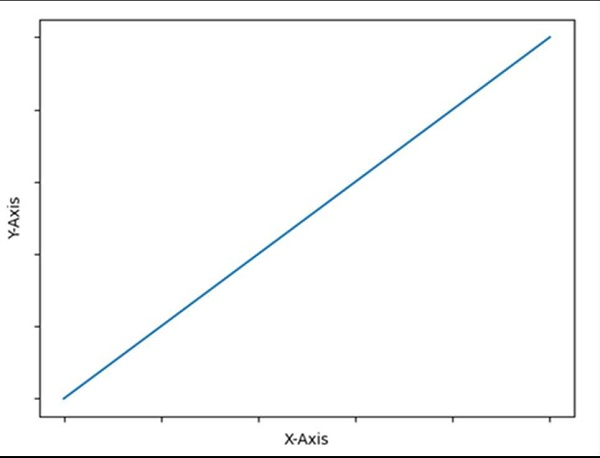# Hide axis values but keep axis tick labels in matplotlib

To hide the axis value but to keep the axis tick labels, we can perform the following steps −

• Plot a line using the plot( ) method.

• Set X and Y labels using x label and y label methods.

• Using plt.gca(), get the current axis, creating one if necessary.

• Use xaxis.set_ticklabels() with an empty list.

• Use yaxis.set_ticklabels() with an empty list.

• To show the diagram, use the plt.show() method.

## Example

import matplotlib.pyplot as plt

plt.plot([0, 5], [0, 5])

plt.ylabel("Y-axis ")
plt.xlabel("X-axis ")

ax = plt.gca()
ax.axes.xaxis.set_ticklabels([])
ax.axes.yaxis.set_ticklabels([])
plt.show()

## Output